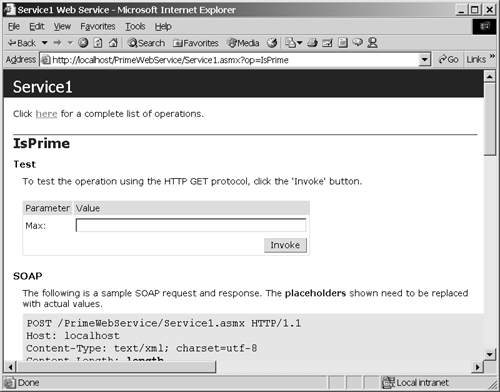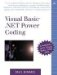# Returning Simple Data from Web Services

Chapter 13 demonstrated the technologies that support Web Services and how to implement a very basic Web Service, the Commissions Web Service. I will pick up from there and show you how to create a Web Service that returns simple data in a practicable way.

Prime numbers ”numbers divisible only by themselves or by the number 1 ”are useful in a tremendous variety of mathematics equations as well as encryption. (Unbreakable encryption relies on huge numbers that are the product of two very large primes. The idea is that by the time any known algorithm can factor the product, the data is no longer relevant.) The Web Service in Listing 14.1 demonstrates how to determine whether a number is prime by using a 2,000-year-old technique devised by Eratosthenes, referred to as the Sieve of Eratosthenes .

You can look up Eratosthenes on the Internet for factual details and biographical information, but I will paraphrase the basic mathematics and theory here for brevity. The Sieve of Eratosthenes works on the premise of the product of primes. The basic idea is that all positive nonprime integers other than 0 and 1 are the products of prime numbers. Eratosthenes further resolved that when trying to determine whether a number is prime, it is necessary to check as possible divisors only those numbers less than or equal to the square root of the candidate number. This makes sense simply because if a candidate number has divisors (other than 1 and itself), one of the divisors will be less than or equal to the square root and the other will be greater than or equal to the square root (or the divisor will be the integer square root). Consider the number 7. The square root of 7 is less than 3 because 3 2 is 9. Thus if 7 had prime divisors (other than 7), the set of divisors would be the integer square root of 7 or one divisor less than 3 and a divisor greater than 3. Since all we need is one divisor (other than 1 or the number itself) to demonstrate that a number is not prime, we would only need to find a divisor less than or equal to the square root of 7.

Eratosthenes resolved that if one knew all the prime numbers preceding any possible prime, the unknown number could be evaluated by checking to see whether any known primes less than the square root were divisors. The basic sieve works by beginning with a known prime (2) and calculating all of the numbers greater than 2 in succession, using all the preceding discovered primes as possible divisors for each number in succession. The algorithm is pretty simple. In the next subsection I will demonstrate my implementation of the Sieve of Eratosthenes as a Web method, and following that subsection I will discuss a simple strategy for implementing Web Services.

#### Implementing the Sieve of Eratosthenes

The basic strategy I employ for all programming is to solve the problem regardless of the way in which I intend a particular consumer to use the solution. Essentially, even though I know I will be presenting a solution as a Web method, I solve the general problem first and then expose the solution for consumers as a Web method.

As a good general strategy, always solve a problem in the general sense as methods and a class, if applicable . For example, one method alone does not yield a very good class, but one method might be the first method in a math library. Here is the promised Listing 14.1, demonstrating my recollected interpretation of the Sieve of Eratosthenes. The listing is followed by a brief summarization.

##### Listing 14.1 A General Solution for Determining Whether a Positive Integer Is Prime
` 1:  Public Class PrimeLibrary 2:    Private FPrimes As ArrayList 3: 4:    Public Sub New(ByVal Max As Long) 5:      FPrimes = New ArrayList() 6:      Seed() 7:      Initialize(Max) 8:    End Sub 9: 10:   Public Function IsPrime(ByVal Number As Long) As Boolean 11:     Return FPrimes.IndexOf(Number) > -1 12:   End Function 13: 14:   Public ReadOnly Property Primes() As ArrayList 15:   Get 16:     Return FPrimes 17:   End Get 18:   End Property 19: 20:   Private Sub Seed() 21:     FPrimes.Add(2L) 22:   End Sub 23: 24:   Private Sub Initialize(ByVal Max As Long) 25:     If (Max < 3) Then Return 26:     Dim I As Long 27:     For I = 3 To Max 28:       If (Test(I)) Then FPrimes.Add(I) 29:     Next 30:   End Sub 31: 32:   Private Function Test(ByVal NumberToTest As Long) As Boolean 33:     Dim Prime As Long 34:     For Each Prime In FPrimes 35:       If (NumberToTest Mod Prime = 0) Then Return False 36:       If (Prime >= Math.Sqrt(NumberToTest)) Then Return True 37:     Next 38: 39:     Return True 40:   End Function 41: 42: End Class `

The general solution is comprised of a class with several methods. The constructor initializes an array to store the list of primes. The IsPrime function and the Primes property represent the public interface to the PrimeLibrary class. The solution works by seeding the ArrayList object with the first known prime, 2, and then calculating all the primes from 3 to the last number we want to test. The number we want to test is represented by the Max argument.

Now that we have a solution that can be used by any application, I can play the role of class consumer and produce the Web Service. The Web Service can expose as much of the class as I'd like, but in this case we will simply expose the IsPrime function. This way we can conceal the complexity of creating the PrimeLibrary class and simply create a fa §ade that provides the result.

#### Implementing the IsPrime Web Service

Visual Studio .NET supports the concept of the solution. Solutions can contain several projects and produce one or more assemblies. PrimeLibrary.sln contains PrimeLibrary.vbproj , a TestPrimeLibrary.vbproj console application (for scaffolding the general solution before building the Web Service), and the Web Service itself.

Chapter 13 described how project templates in the New Project dialog can create all the basic source code for an ASP.NET Web Service by using the template code. Because the template exists, we only have to worry about implementing our Web methods. Listing 14.2 contains the implementation of PrimeWebService.vbproj .

## NOTE

You can create your own project templates. To create a new item or project template, copy an existing template that is close to the new template you want to create. Modify the existing template files to contain the code your template will add, create a Wizard Launching file ( .vsz ), and add an entry to a .VSDir file. For more information, look in the VS .NET help documentation.

##### Listing 14.2 A Web Service That Uses the PrimeLibrary Class
` 1:  Imports System.Web.Services 2:  Imports Library = PrimeLibrary.PrimeLibrary 3: 4: 5:  <WebService(Namespace:="http://tempuri.org/")> _ 6:  Public Class Service1 7:    Inherits System.Web.Services.WebService 8: 9:    [ Web Services Designer generated code ] 10:   <WebMethod()> Public Function IsPrime( _ 11:     ByVal Max As Long) As Boolean 12: 13:     Dim Instance As Library = New Library(Max) 14:     Return Instance.IsPrime(Max) 15: 16: End Function 17: 18: End Class `

This Web Service is about as straightforward as it can be. PrimeWebService.vbproj implements one Web Service using the default name Service1 . I added a reference to PrimeLibrary.vbproj , which in turn gives me access to the PrimeLibrary class and the general solution for the prime test. In line 2 I used the aliasing technique that lets us substitute a local namespace alias for a long namespace and class reference. It just happens that my namespace and class are both named PrimeLibrary . Using the aliasing technique in line 2, I shortened the whole thing to just Library .

The single Web method is implemented in lines 10 through 16. The WebMethod attribute indicates that IsPrime will be callable from clients across a network. The two statements simply create an instance of the PrimeLibrary class using the alias and return the result of PrimeLibrary.IsPrime . Figure 14.1 shows the IsPrime Web Service accessible from the .asmx -generated test page.

##### Figure 14.1. Access the IsPrime Web Service by browsing to the .asmx page.This kind of Web Service supports the idea of Web Services as a way to return simple data. You will probably more often want to return complex data types. Complex data can be returned in the form of typed collections, instances of a class, or DataSet objects. The next section demonstrates how to return complex data from a Web Service.Visual Basic(R) .NET Power Coding
ISBN: 0672324075
EAN: 2147483647
Year: 2005
Pages: 215
Authors: Paul Kimmel